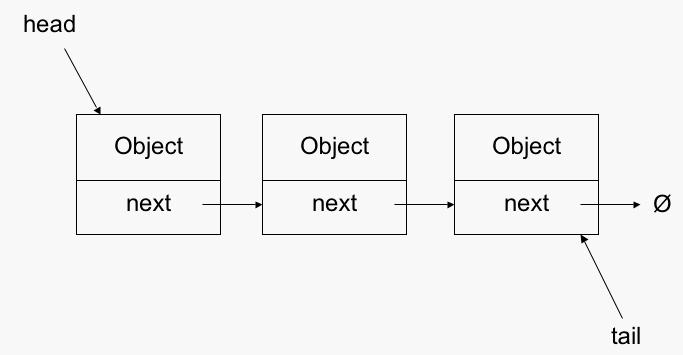• Linked lists are a way to store data with structures so that the programmer can automatically create a new place to store data whenever necessary.
• Specifically, the programmer writes a struct definition that contains variables holding information about something and that has a pointer to a struct of its same type.Example 1
Creating a linked list. Function malloc is used to allocate memory space for the structure.

```#include <stdio.h>
#include <stdlib.h>
struct node
{
int item;
struct node *next;
};

int main()
{
struct node *root; /* This will be the unchanging first node */

/* This will point to each node as it traverses the list */
struct node *conductor;

//allocating space for the root
root = malloc(sizeof(struct node));

root->next = 0;
root->item = 777;

conductor = root;

int x;

//create ten new nodes

for(x = 1; x <=10; ++x)
{
/* Creates a node at the end of the list */
conductor->next = malloc( sizeof(struct node) );

/*checking that memory is allocated*/
if ( conductor == 0 )
{
printf( "Out of memory" );
return 0;
}

conductor = conductor->next;
conductor->item = x;
}

//marking the last as NULL
conductor->next = 0;

//conductor points to root again
conductor = root;

//print the list
if ( conductor != 0 )  /* Makes sure there is a place to start */
{
while(conductor->next != 0)
{
printf(" [%d]-> ",conductor->item);
conductor = conductor->next;
}
}

getchar();

}
```